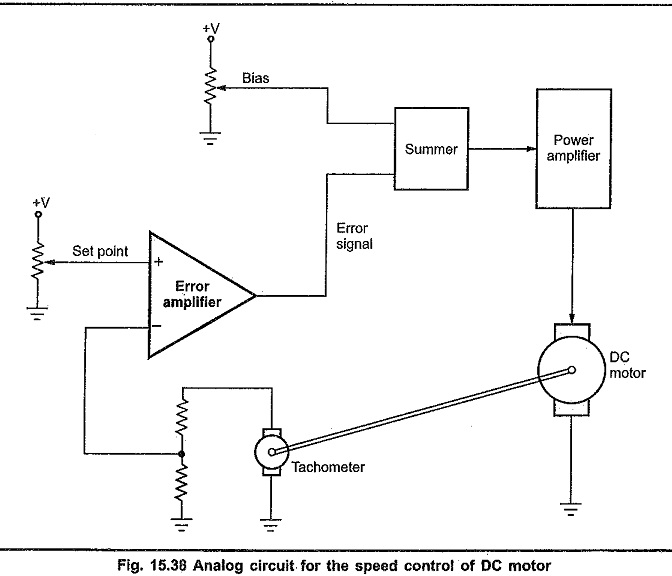# Speed Control of DC Motor

## Speed Control of DC Motor:

The Fig. 15.38 shows the analog approach to control the Speed Control of DC Motor. A tachometer is used to get the information about the Speed Control of DC Motor. It is a DC generator mounted on the shaft of the DC motor. It gives the output voltage proportional to the speed of the motor. The output voltage is typically a few volts per 1000 rpm. A fraction of the output voltage from the tachometer is feedback to the inverting input of the error amplifier.

A positive voltage is applied to the non inverting input of the error amplifier as a set point. The output of error amplifier and bias voltage is added using summer circuit and it them amplified by the power amplifier. The output of power amplifier drives the DC motor.At normal condition, motor rotates at constant speed set by the set point and bias inputs. When motor rotates at set speed, the set point voltage and the fraction of voltage fed back to inverting terminal are same. Therefore, the differential input to the error amplifier is zero, resulting zero output. In this case only bias voltage is amplified applied to the DC motor. This amplified bias voltage is necessary to rotate motor at set speed.

If the speed of the motor decreases due to extra load on the motor, the output voltage from the tachometer decreases and it is now less than the setpoint voltage. This difference in two voltages is amplified by the error amplifier to produce error signal. As set point voltage is greater than tachometer voltage, error is positive and it is added to the bias voltage by summer circuit.

The power amplifier, amplifies the increased output of summer circuit to give more excitation to the DC motor. With increased excitation, motor speed increases and it approaches to set speed.

On the other hand, when the speed of DC motor increases due to less load, the tachometer voltage increases. Now, tachometer voltage is greater than the set point voltage. This produces negative error voltage. Due to negative error voltage, summer circuit actually subtracts the error voltage from the bias voltage.

The power amplifier, amplifies the decreased output of the summer circuit to give less excitation to the DC motor. Less excitation results slower speed of DC motor. Therefore, the increased speed is compensated by reduced speed of DC motor.

The above discussed method is called proportional control. Because the output of power amplifier is proportional to the error signal